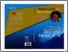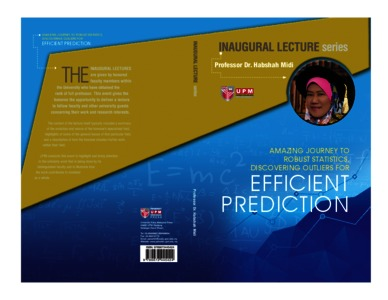Simple Search:

Amazing journey to robust statistics, discovering outliers for efficient prediction

Citation

Midi, Habshah (2016) Amazing journey to robust statistics, discovering outliers for efficient prediction. [Inaugural Lecture]

Abstract / Synopsis

In today’s society, statistical techniques are being used widely in education, medicine, social sciences, and applied sciences. They are crucial in interpreting data and making decisions. When one makes a statistical inference, it is very crucial to be aware of the assumptions under which the statistical testing procedures can be applied. The assumptions that are common to almost all statistical tests are that the observations are random, independent and identically distributed, come from a normal distribution and they are equally reliable and should have equal role in determining the results. The last assumption implicitly states that there is no outlier in a data set. Outliers are observations which are markedly different or far from the majority of observations. In most statistical models, the assumptions of normality of errors, no multicollinearity, homoscedasticity and non-autocorrelated errors are often violated. Another assumption that has received much attention from statisticians in recent years is that the regression analysis must be free from the effect of outliers. Even though the Ordinary Least Squares (OLS) estimates retain unbiasedness in the presence of heteroscedasticity, multicollinearity and autocorrelation, their estimates become inefficient. As such, proper diagnostic checking should first be considered before further data analysis is carried out. The problem gets more complicated, when the violation of homoscedasticity, no multicollinearity, and no autocorrelation, each comes together with the existence of outliers. Methods that are designed to rectify these problems, cannot handle both problems simultaneously. In this regard, proper remedial measures should be taken into consideration to remedy these problems. Hence, some robust methods which are developed to simultaneously remedy these two problems will be illustrated in this inaugural lecture. Robust method is a relatively new method whereby it is not easily affected by outliers because their effects are reduced. This presentation also focuses on our research, in developing robust diagnostic methods for detecting whether or not outliers, multicollinearity, heteroscedasticity and autocorrelated errors are present in a data. This presentation also will illustrate some of our developed diagnostic methods to identify high leverage points and also to indicate whether multicollinearity is caused by correlated predictors or high leverage points. This presentation will also illustrates the effects of outliers and high leverage points on panel data model, response surface model and variable selection methods. Outliers are known to have an adverse effect on computed values of various estimates. The immediate consequences of outlier are that they may cause apparent non-normality and the entire classical methods breakdown. Classical methods heavily depend on assumptions. However, in practice those assumptions are difficult to be met. Violations of at least one of the assumptions may produce suboptimal or even invalid inferential statements and inaccurate predictions. Since outliers give bad consequences, the need for robust methods become essential to avoid misleading conclusion. Hence, we developed several robust methods pertaining to these issues. Due to space limitations, only some selected developed robust methods will be presented in this inaugural lecture and their mathematical derivations are not shown.Preview
PDF
20170727170159Amazing_Journey_Robust_Statistics_Discovering_Outlier_for_Efficient_Prediction.pdfView Item